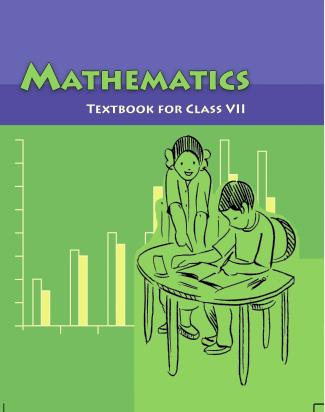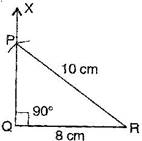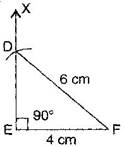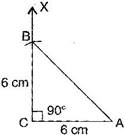1. /
2. CBSE
3. /
4. Class 07
5. /
6. Mathematics
7. /
8. NCERT Solutions for Class...

# NCERT Solutions for Class 7 Maths Exercise 10.5### myCBSEguide App

Download the app to get CBSE Sample Papers 2023-24, NCERT Solutions (Revised), Most Important Questions, Previous Year Question Bank, Mock Tests, and Detailed Notes.

NCERT solutions for Maths Practical Geometry## NCERT Solutions for Class 7 Maths Practical Geometry

###### Question 1.Construct the right angled {tex}\Delta {/tex}PQR, where {tex}m\angle {/tex}Q = {tex}{90^ \circ },{/tex} QR = 8 cm and PR = 10 cm.

To construct: A right angled triangle PQR where {tex}m\angle {/tex}Q = {tex}{90^ \circ },{/tex} QR = 8 cm and PQ = 10 cm.Steps of construction:

(a) Draw a line segment QR = 8 cm.

(b) At point Q, draw QX {tex} \bot {/tex} QR.

(c) Taking R as centre, draw an arc of radius 10 cm.

(d) This arc cuts QX at point P.

(e) Join PQ.

It is the required right angled triangle PQR.

NCERT Solutions for Class 7 Maths Exercise 10.5

###### Question 2.Construct a right angled triangle whose hypotenuse is 6 cm long and one the legs is 4 cm long.

To construct: A right angled triangle DEF where DF = 6 cm and EF = 4 cmSteps of construction:

(a) Draw a line segment EF = 4 cm.

(b) At point Q, draw EX {tex} \bot {/tex} EF.

(c) Taking F as centre and radius 6 cm, draw an arc. (Hypotenuse)

(d) This arc cuts the EX at point D.

(e) Join DF.

It is the required right angled triangle DEF.

NCERT Solutions for Class 7 Maths Exercise 10.5

###### Question 3.Construct an isosceles right angled triangle ABC, where {tex}m\angle {/tex}ACB = {tex}{90^ \circ }{/tex} and AC = 6 cm.

To construct: An isosceles right angled triangle ABC where {tex}m\angle {/tex}C = {tex}{90^ \circ },{/tex} AC = BC = 6 cm.Steps of construction:

(a) Draw a line segment AC = 6 cm.

(b) At point C, draw XC {tex} \bot {/tex} CA.

(c) Taking C as centre and radius 6 cm, draw an arc.

(d) This arc cuts CX at point B.

(e) Join BA.

It is the required isosceles right angled triangle ABC.

## NCERT Solutions for Class 7 Maths Exercise 10.5

NCERT Solutions Class 7 Maths PDF (Download) Free from myCBSEguide app and myCBSEguide website. Ncert solution class 7 Maths includes text book solutions from Class 7 Maths Book . NCERT Solutions for CBSE Class 7 Maths have total 15 chapters. 7 Maths NCERT Solutions in PDF for free Download on our website. Ncert Maths class 7 solutions PDF and Maths ncert class 7 PDF solutions with latest modifications and as per the latest CBSE syllabus are only available in myCBSEguide.

## CBSE app for Students

To download NCERT Solutions for Class 7 Maths, Social Science Computer Science, Home Science, Hindi English, Maths Science do check myCBSEguide app or website. myCBSEguide provides sample papers with solution, test papers for chapter-wise practice, NCERT solutions, NCERT Exemplar solutions, quick revision notes for ready reference, CBSE guess papers and CBSE important question papers. Sample Paper all are made available through the best app for CBSE students and myCBSEguide website.Test Generator

Create question paper PDF and online tests with your own name & logo in minutes.myCBSEguide

Question Bank, Mock Tests, Exam Papers, NCERT Solutions, Sample Papers, Notes

### 3 thoughts on “NCERT Solutions for Class 7 Maths Exercise 10.5”

1. So good

2. Super

3. Thanks
You are solve my problems this exercise